SAMPLE SALE NOW ON ... DON'T MISS OUT!

Not finding the mirror you are looking for? Let us create it for you! It is very easy.

.

.

1.  S E L E C T   T H E   M I R R O R   T I N T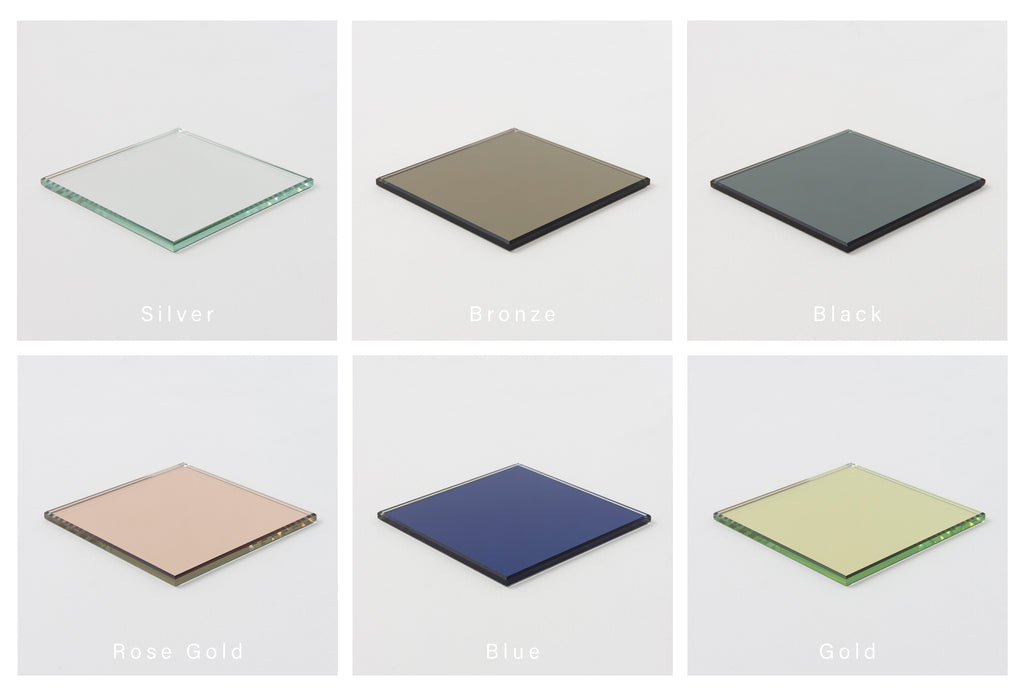.
.
A n t i q u e d    F i n i s h e s.

2.  S E L E C T   T H E   S I Z E   A N D  S H A P E

Round    I    Capsula     I    Oval    I    Arched    I    Square    I    Rectangular    I    Half Moon  &  more ...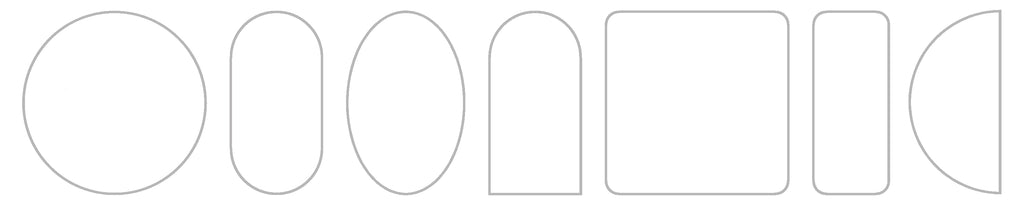.

.

3.  S E L E C T    F R A M E    C O L O U R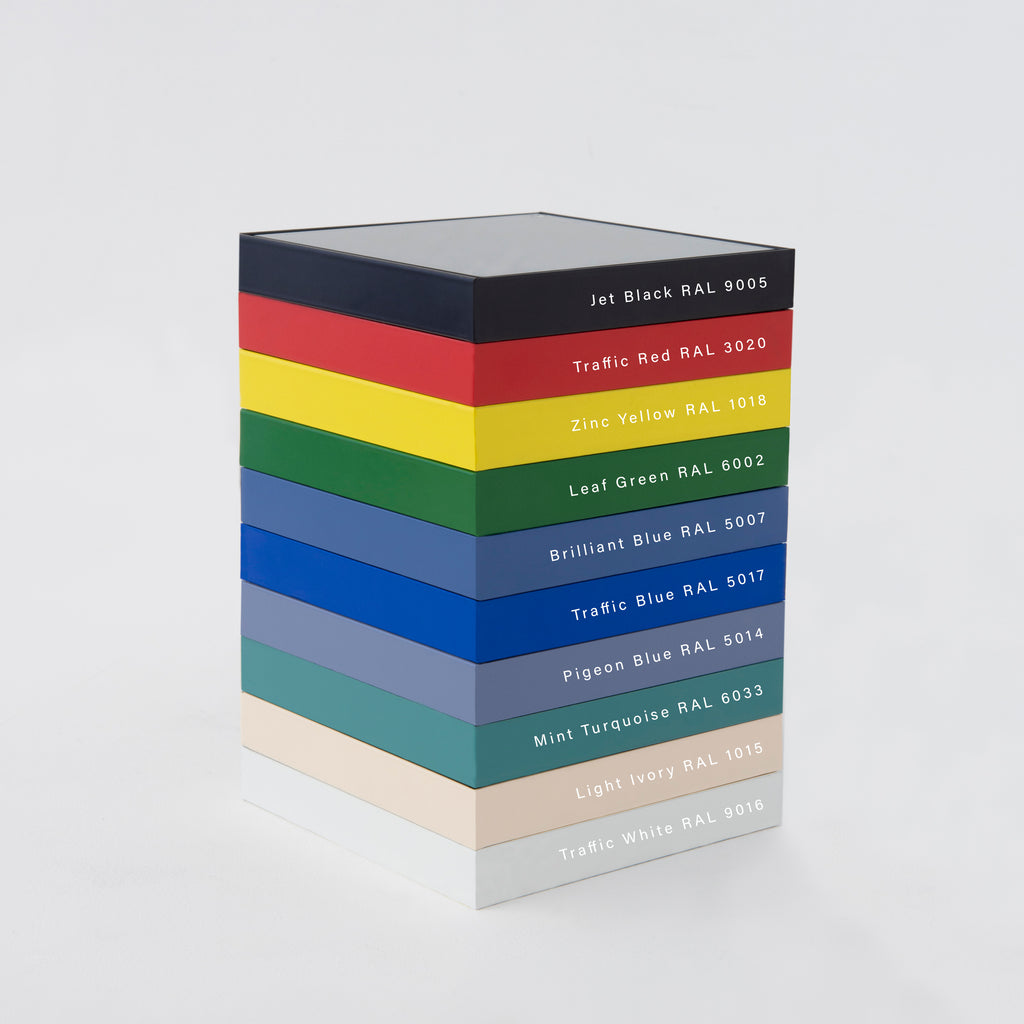.

o r

S P E C I F Y    F R A M E    M A T E R I A L

B r a s s   (Brushed, Polished or with a Bronze Patina)
S t a i n l e s s   S t e e l (Brushed, Polished or Blackened)
C o p p e r   (Brushed or Polished)
N i c k e l  P l a t e dm i r r o r   d e p t h  o p t i o n s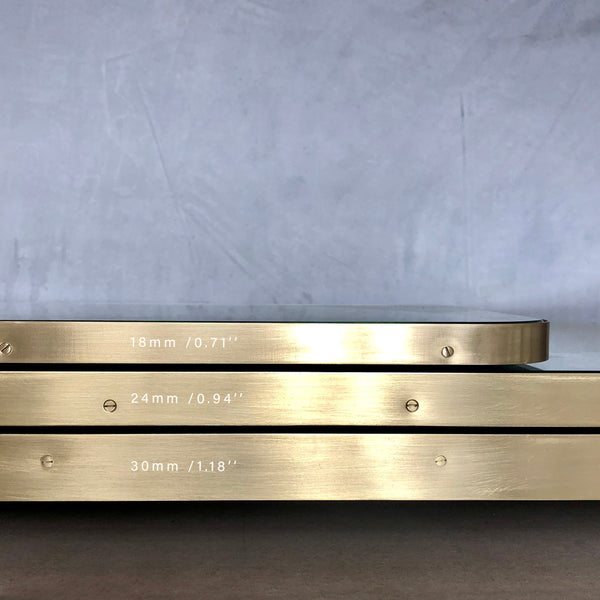o r

F R A M E L E S S

.

.

4.  S E L E C T   M I R R O R   E X T R A S  (if required)

B e v e l l e dS u s p e n d e d    |    Front  or  Back  I l l u m i n a t e d    I    D e m i s t e r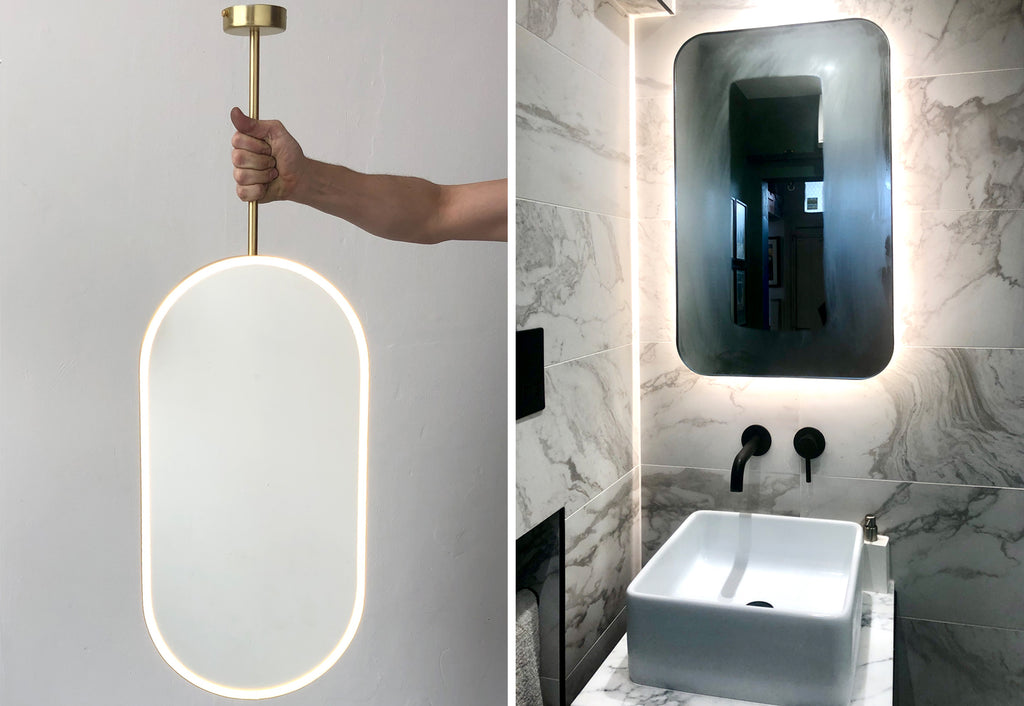5.  C O N T A C T    U S  !